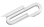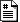Fall 2018

# Thu Oct 11

Discuss the material in chapter 8.

## more ways to loop

• "while" loops
• "sentinel" pattern of input or file processing
• "break" from the middle of a loop (... or put it in a function and use "return")
• "while True:" construct
• nested loops
• type conversions to boolean

See the attached average4a.py as an example of some of these ideas.

## boolean algebra

• and, or, not
• De Morgan's Laws
• WRONG: if answer == 'y' or 'Y'
• "implies" operator ... what is its truth table? Can you build something equivalent from (and, or, not) ?
(not x) and (not y)    # which of these are the same ?
not (x and y)
not (x or y)


Well ... let's check.

layout = " {:6}  {:6}   {:16}  {:16} "

print(layout.format('a', 'b', 'not (a and b)', '(not a) or (not b)'))
print(layout.format('-'*6,'-'*6, '-'*16, '-'*16))

for a in (True, False):
for b in (True, False):
print(layout.format(str(a),
str(b),
str(not (a and b)),
str((not a) or (not b))))


which outputs this

 a       b        not (a and b)     (not a) or (not b)
------  ------   ----------------  ----------------
True    True     False             False
True    False    True              True
False   True     True              True


In the next homework I'm going to ask you to do something similar for DeMorgan's laws.

## short-circuit evaluation

def t():
print("in t")
return True

def f():
print("in f")
return False

for expression in ['f() and t()',
't() and f()',
'f() or t()',
't() or f()']:
print('-- {} --'.format(expression))
print(eval(expression))


which produces

-- f() and t() --
in f
False
-- t() and f() --
in t
in f
False
-- f() or t() --
in f
in t
True
-- t() or f() --
in t
True


The first and last case terminate without making the second function call. These constructions can be used to accomplish something like an "if" construction, in the sense of "We better leave now or we'll miss our train."

In the first case, since f() is false, (f() and t()) must be false, so t() isn't evaluated.

In the last case, since t() is true, (t() or f()) must be true, and so t() isn't evaluated.

An example often used in the perl programming language in constructions like "open(filename) or die('cannot open file')", in which open() returns False if it cannot, and die() is a built-in that prints an error message and exits the program.

## Lewis Carroll puzzles

One amusing example of the sort of logic we're doing this week are Lewis Carroll puzzles ...

(1) Every one who is sane can do Logic;
(2) No lunatics are fit to serve on a jury;
(3) None of your sons can do Logic.
What conclusion can be drawn?
Use
a = able to do Logic
b = fit to serve on a jury
c = sane


Express each line as a boolean expression. Then use the rules of boolean algebra to draw a short conclusion.

Answer: None of your sons is fit to serve on a jury.

How can we do that using logic rules? python??

https://cs.marlboro.college /cours /fall2018 /python /notes /chap8
## attachmentslast modified sizeaverage4a.py Tue Dec 05 2023 09:04 am 908B